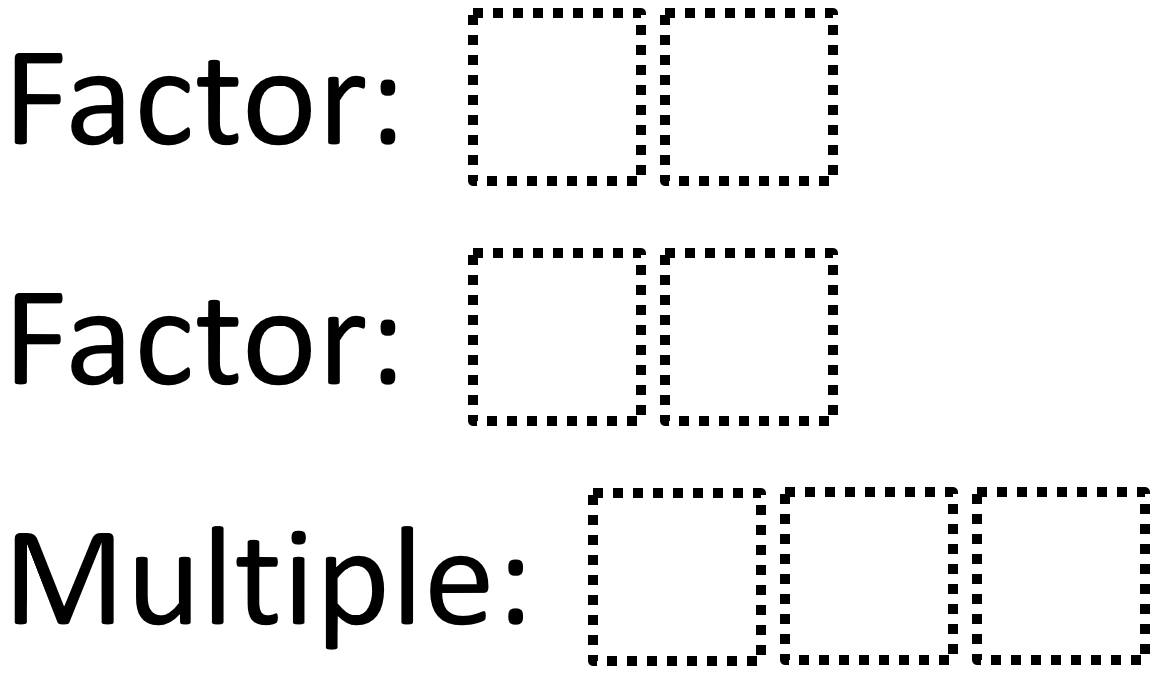Home > Grade 6 > Least Common Multiple

# Least Common Multiple

Directions: Use the digits 0 to 9, at most one time each, to fill in the boxes and make a true statement.### Hint

How does choosing the digit for the ones’ place affect the least common multiple?

One answer is LCM 28 and 35 is 140. There may be others.

Source: Wendy Taylor

## Largest Possible GCF #2

Directions: Using the digits 0-9 at most once, fill in the boxes to make the …

1.Another is LCM of 07 and 24 is 168

2.We found 52 x 09 = 468

3.LCM of 02 and 19 is 38

•You need the multiple to be a 3 digit number, so this would not work because you would use zero twice.

4.•same as above

5.LCM of 36 and 45 is 180.